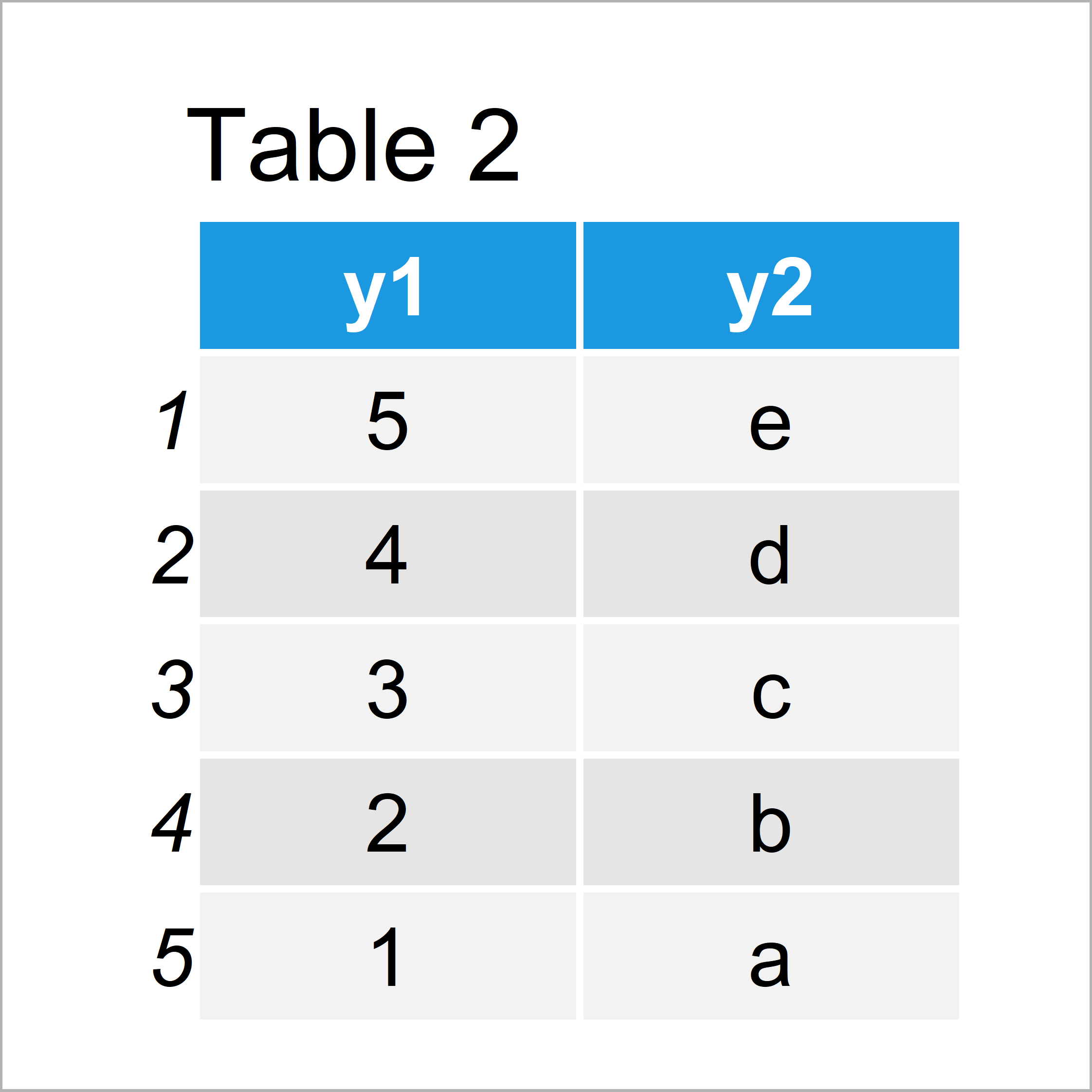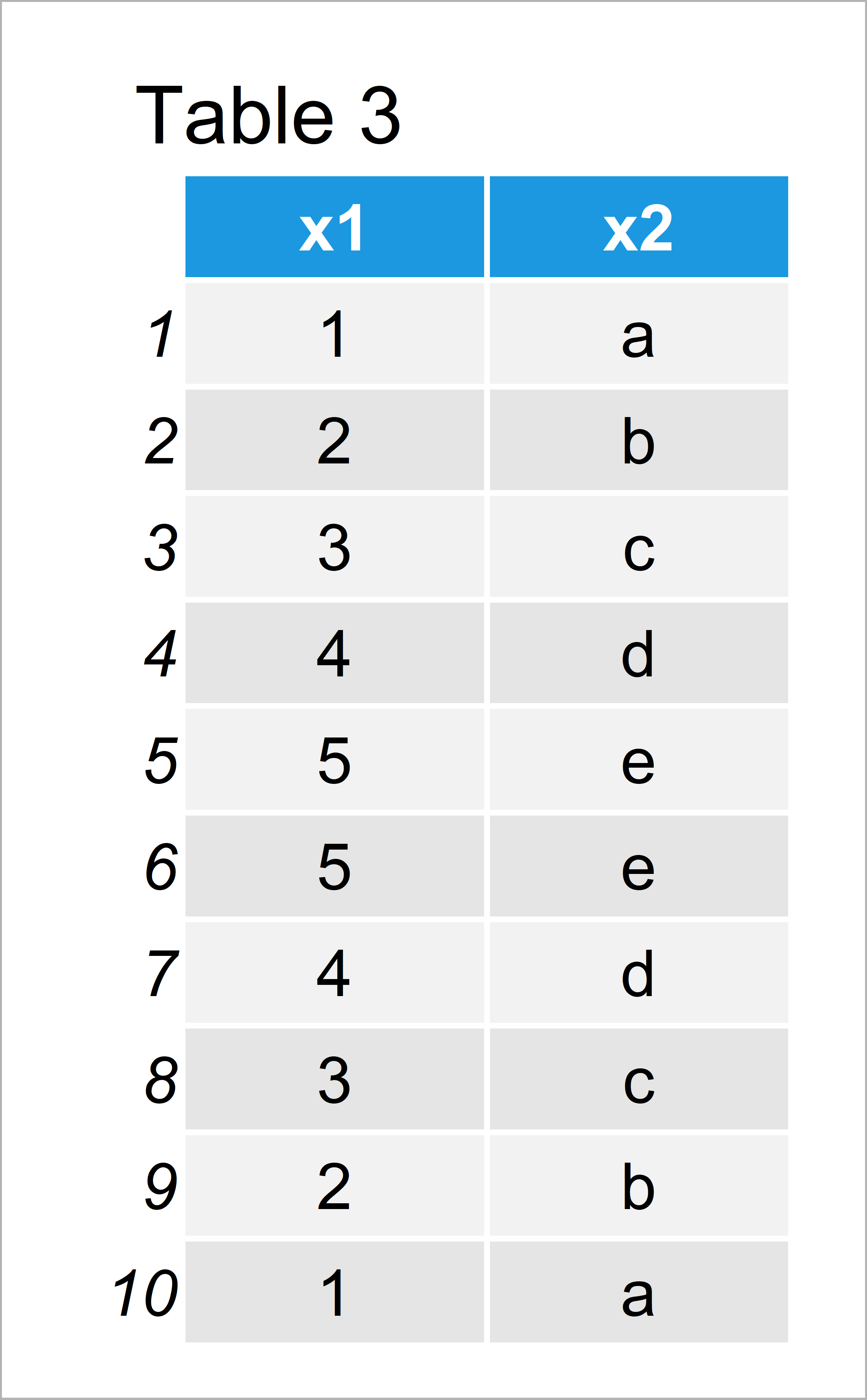# rbind Data Frames by Column Index in R (Example)

In this tutorial you’ll learn how to ignore variable names when applying the rbind() function in R programming.

Sound good? Great, let’s just jump right in…

## Example Data

Consider the following example data frame:

```data1 <- data.frame(x1 = 1:5,    # Create first data frame
x2 = letters[1:5])
data1                            # Print first data frame```As you can see based on Table 1, our first example data is a data frame containing five rows and the two variables x1 and x2.

Let’s create another data frame in R:

```data2 <- data.frame(y1 = 5:1,    # Create second data frame
y2 = letters[5:1])
data2                            # Print second data frame```Table 2 visualizes the output of the previous code: Our second data frame also consists of five rows and the columns. However, the variables are called differently (i.e. y1 and y2).

If we now try to concatenate our data frames using the rbind function, the following error message is returned by the RStudio console:

```data_all <- rbind(data1, data2)  # rbind returns error message
# Error in match.names(clabs, names(xi)) :
#   names do not match previous names```

So how can we avoid this error message and append the second data frame to the first data frame based on the index positions of our variables?

## Example: Ignore Column Names when Using rbind() Function

In this example, I’ll illustrate how to bind data frames by index positions instead of variable names.

For this, we have to use the setNames and names functions to temporarily rename the column names of our second data frame.

Have a look at the following R syntax:

```data_all <- rbind(data1,         # Rename columns & rbind
setNames(data2, names(data1)))
data_all                         # Print row-binded data frame```After running the previous R code the combined data frame shown in Table 3 has been created. As you can see, we managed to bind our two data frames together, even though the column names were different.

Please note that this should be done with care! Always makes sure that it makes theoretical sense when combining data frames.

## Video, Further Resources & Summary

Do you want to know more about row-binding and merging data? Then you might watch the following video of my YouTube channel. I’m illustrating the R syntax of this tutorial in the video tutorial.

Please accept YouTube cookies to play this video. By accepting you will be accessing content from YouTube, a service provided by an external third party.If you accept this notice, your choice will be saved and the page will refresh.

Furthermore, you might want to read the related articles of https://statisticsglobe.com/.

You have learned in this tutorial how to row-bind data frames by the column index in R programming. In case you have additional questions, don’t hesitate to let me know in the comments section.

Subscribe to the Statistics Globe Newsletter